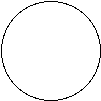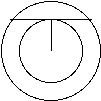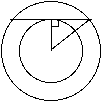×#### Thank you for registering.

One of our academic counsellors will contact you within 1 working day.

Click to Chat

1800-1023-196

+91-120-4616500

CART 0

• 0

MY CART (5)

Use Coupon: CART20 and get 20% off on all online Study Material

ITEM
DETAILS
MRP
DISCOUNT
FINAL PRICE
Total Price: Rs.

There are no items in this cart.
Continue Shopping
```
how do you work out the perimeter of a circle which is in a larger one and a chord is given on the tangent to the smaller circle

```
6 years ago

```							The best way to go about a problem like this is to create a diagram of what you are actually trying to figure out. So you are starting with a circleThen we have a circle inside of this circle and since the size of the smaller circle can change we will give this circle a radiusr.Now the toughest part is placing the chord. It says that the chord rests on top of the smaller circle so we can place it anywhere.A theorem in geometry is that if a chord touches the edge of a circle it creates a right angle with the radius of that circle.Then from here we can use the Pythagorean Theorem to help us find the radius of the big circle. Since the length of the chord is x half of that would be 50 and this would be one of the legs of the right triangle, then the radiusrwould be the length of the other leg of the right triangle. So your equation for the radius R of the larger circle can be figured out using the Pythagorean Theorem and looks like the following equation R2= r2+x2. Thus i you know the radius r of the smaller circle you can find the radius R of the larger circles radius you then can use A=pR2o find the area of the larger circle.Hope this helps.Thank YouRuchiAskiitians Faculty
```
6 years ago
Think You Can Provide A Better Answer ?

## Other Related Questions on 10 grade maths

View all Questions »### Course Features

• 728 Video Lectures
• Revision Notes
• Previous Year Papers
• Mind Map
• Study Planner
• NCERT Solutions
• Discussion Forum
• Test paper with Video Solution### Course Features

• 731 Video Lectures
• Revision Notes
• Test paper with Video Solution
• Mind Map
• Study Planner
• NCERT Solutions
• Discussion Forum
• Previous Year Exam Questions